# Issue with weighting when importing displacements

Hi,

I have data for the displacements of 48 nodes on a cross-section of a brain and I need to determine strain. The data is in a CSV which is organized into columns like this: 1st column is x displacements for node 1, 2nd columns is z displacements for node 1, 3rd columns is x for node 2 and so on. There are no displacements in the y direction. I imported the CSV as external data and created the necessary named selections on the model. The issue is when I try to assign the imported displacements to the named selection there's an error with weighting and I can't solve. Here's a picture of what it all looks like: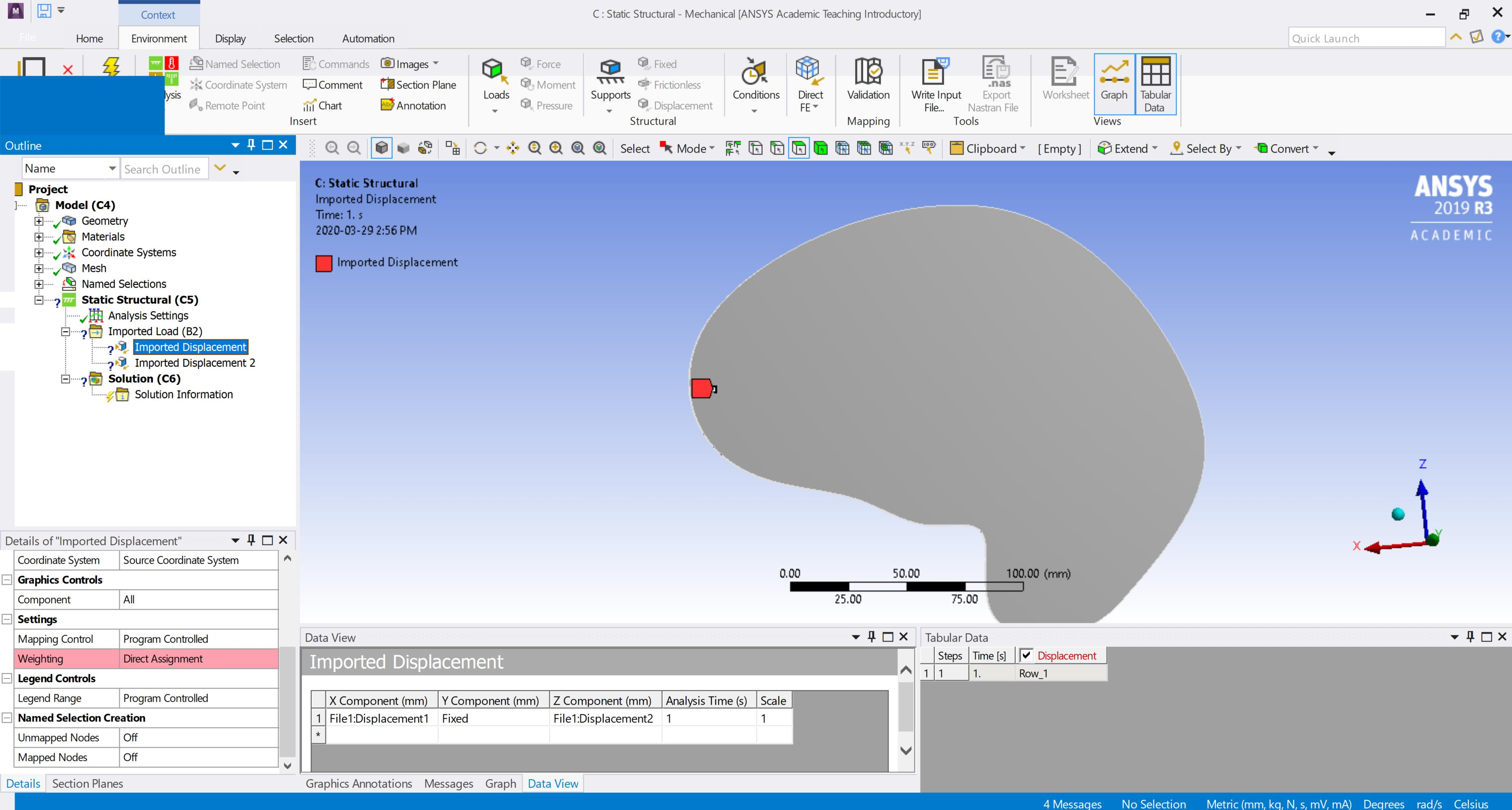I would greatly appreciate it if someone could let me know what the issue here is, thank you!

• Also need the X,Z coordinates of each node. If you have a mesh that connects these nodes as triangular elements, that could also be useful.

• I have a CSV with all the coordinates too, do I need to import that as well? How would I go about adding the coordinates to the named selections?

• In Workbench, drag out a Static Structural analysis. Click on the Geometry cell and in the Properties on the right, set the Analysis Type to 2D. Open SpaceClaim. Create points using the coordinates. Call the Z coordinate a Y coordinate so you can do the problem in 2D and the points will be on the XY plane. Connect the points with lines to form triangles. The triangles will form surfaces. At the end, you will have a surface body with several faces. Do you have matlab?  A function called delaunay can compute how to draw triangles through a set of points. If you don't have matlab, put the .CSV file in a .zip file and attach it after you reply.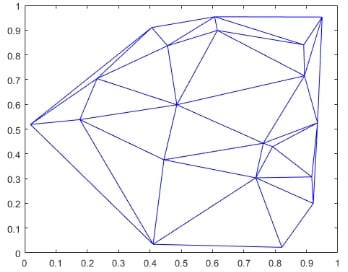You don't have to use triangles, if 4-sided objects fit the points nicely, you can use those too. You can have 5 or 6 sided faces too.

Once you have a surface with a set of vertices that match the coordinates of the points, you take it into Mechanical and mesh it. Now you can assign a Displacement to each of the 48 vertices with the displacement data you have.

Solve and the surface will deform to have a continuous displacement field.

• Sorry, if iam jumping to another problem.

I want to create the "imported Displacement" by ACT console python Script.

I tryed below.. This is not working

analysis = ExtAPI.DataModel.Project.Model.Analyses

imported = [i for i in analysis.Children if 'Imported Loads' in i.Name]

............

I tryed this too

iam getting errorr : 'List[DataModelObject]' object has no attribute 'AddImportedDisplacement'

• Sorry, I don't work with python code. Maybe someone else can help.

• Hi, sorry for the late response and thanks for the help!

The geometry is more complex then just the point cloud, therefore I imported the geometry first and the point cloud on top of it to SpaceClaim and then created named selections for each point. I'm doing it in 3d because the geometry I was given does have a slight thickness to it. The nodes seem to be in their proper positions on the geometry but I still have that weighting error when assigning displacements in Mechanical. Does the error have something to do with the way I'm importing the point cloud to SpaceClaim?

• Change the 3D geometry to a 2D model.

In SpaceClaim

• make a plane and slice through the solid geometry you were given,
• delete one side then you have a face in the YZ plane.
• Select the face in the YZ plane and Ctrl-C and Ctrl-V.  Now you have a surface.
• Delete the solid.
• Import the Points
• Use a sketch to divide the surface into faces so that each point becomes a vertex of a face.
• Use the Rotate Tool to rotate that surface about the origin and the Y axis by 90 degrees. Now the geometry is in the XY plane.

In Workbench

• Select Geometry cell and change Analysis Type to 2D. You can only do this on a new Static Structural model.

In Mechanical

• Don't import the displacements as an imported load.
• Create a Displacement Load on each vertex and enter the displacement value where Z=X now.

• This should work thank you so much!

Is there a way to insert a table of displacements in Mechanical? It always seems to just copy one row.

• If you were in a Transient Structural, and had a time history of displacement of a point with values over many rows including a first column for Time in seconds, then you can paste 3 columns: Time, X, Y for as many rows as you have.

If you are in a Static Structural model with only one Step, then there is only one row.  You can get a second row if you add a second step.

• I don't have a time history but I do have over 400 displacements. Would my best bet be to use transient structural with an arbitrary time increment for each displacement?

• In a Static Structural model, open Mechanical, Under Analysis Settings, Number of Steps, type 400.
Each step is assigned 1 second, so step 400 is at 400 seconds.

Insert a Displacement, scope it to one of the 48 nodes.  Set the X and Y components to Tabular Data.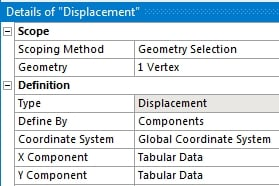Click on the grey square above row 1 to the left of the the word Steps, that will turn the whole table blue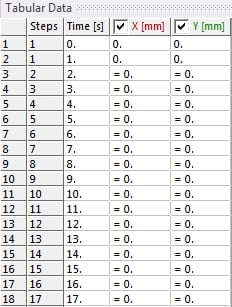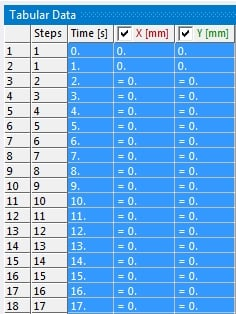In Excel, Copy the three columns by 400 rows of numbers only, then Paste them into the Tabular Data window.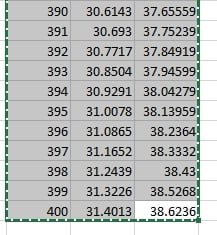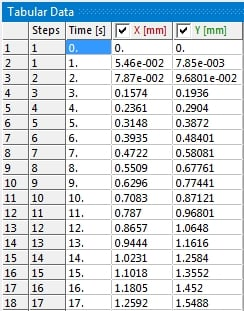Repeat for the other 47 points.

• edited March 2020

Perfect, that should work! Thank you for all your help, cheers!